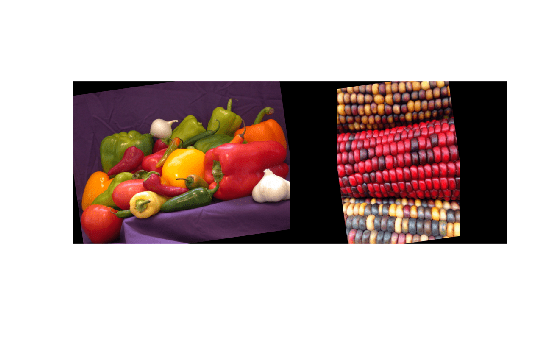Main Content

# augment

Apply identical random transformations to multiple images

## Syntax

``augI = augment(augmenter,I)``

## Description

example

````augI = augment(augmenter,I)` augments image `I` using a random transformation from the set of image preprocessing options defined by image data augmenter, `augmenter`. If `I` consists of multiple images, then `augment` applies an identical transformation to all images.```

## Examples

collapse all

Create an image augmenter that rotates images by a random angle. To use a custom range of valid rotation angles, you can specify a function handle when you create the augmenter. This example specifies a function called `myrange` (defined at the end of the example) that selects an angle from within two disjoint intervals.

`imageAugmenter = imageDataAugmenter('RandRotation',@myrange);`

Read multiple images into the workspace, and display the images.

```img1 = imread('peppers.png'); img2 = imread('corn.tif',2); inImg = imtile({img1,img2}); imshow(inImg)```Augment the images with identical augmentations. The randomly selected rotation angle is returned in a temporary variable, `angle`.

`outCellArray = augment(imageAugmenter,{img1,img2});`
```angle = 8.1158 ```

View the augmented images.

```outImg = imtile(outCellArray); imshow(outImg);```Supporting Function

This example defines the `myrange` function that first randomly selects one of two intervals (-10, 10) and (170, 190) with equal probability. Within the selected interval, the function returns a single random number from a uniform distribution.

```function angle = myrange() if randi([0 1],1) a = -10; b = 10; else a = 170; b = 190; end angle = a + (b-a).*rand(1) end```

## Input Arguments

collapse all

Augmentation options, specified as an `imageDataAugmenter` object.

Images to augment, specified as one of the following.

• Numeric array, representing a single grayscale or color image.

• Cell array of numeric and categorical images. Images can be different sizes and types.

## Output Arguments

collapse all

Augmented images, returned as a numeric array or cell array of numeric and categorical images, consistent with the format of the input images `I`.

## Tips

• You can use the `augment` function to preview the transformations applied to sample images.

• To perform image augmentation during training, create an `augmentedImageDatastore` and specify preprocessing options by using the `'DataAugmentation'` name-value pair with an `imageDataAugmenter`. The augmented image datastore automatically applies random transformations to the training data.

## See Also

### Topics

Introduced in R2018b

Download ebook